# Building Models with Recursive Partitioning Trees, Random Forest, and AdaBoost in R

In Machine Learning & AI, Software Engineering

This is the first homework assignment for Biostatistics 273: Classification and Regression Trees, that is meant as a brief exercise in building models with rpart, randomForests, and AdaBoost in R. The first version of this post will be the content I submitted. I anticipate later versions that will include results from further “playing” with the models and code.

This homework implements rpart (single tree partitioning), randomForests, and AdaBoost to respectively build predictive models for the “sonar” dataset. Predictive models were built with a provided “sonar” training dataset, and misclassification error for each model were evaluated with a “sonar” test dataset. Errors from each procedure are summarized in a table at the end of this report. Training and test datasets were provided by Prof. Kitchen. R 2.15.0 was used with the packages rpart (3.1-52) and randomForest (4.6-7).

This homework also performs a thought experiment, in which multiple ‘seed’ and ‘mtry’ values were used for randomForests.

## 1. Recursive Partitioning with rpart

With its default parameters, rpart produces

```> printcp(rpart_fit)
Classification tree:
rpart(formula = y_train ~ ., data = x_train, method = "class")

Variables actually used in tree construction:
 V11 V27 V52 V54 V8

Root node error: 64/130 = 0.49231

n= 130

CP nsplit rel error  xerror     xstd
1 0.546875      0   1.00000 1.20312 0.087545
2 0.062500      1   0.45312 0.48438 0.075918
3 0.046875      3   0.32812 0.60938 0.081640
4 0.010000      5   0.23438 0.68750 0.084299```

There are four splits that result in five terminal nodes. This can be better visualized with a plot.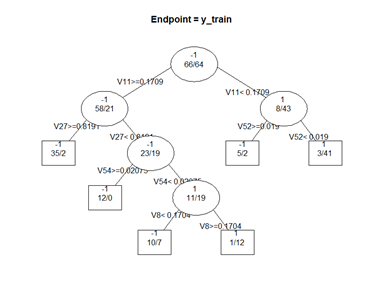From the complexity parameter table, we note that the cross-validation error (“xerror”) decreases from 1.2 to 0.48 with the first split, but increases to 0.61 with the second split. This error is only noticeable portion of the rpart algorithm that is random, and may change with seed number; the resulting tree remains the same, given the same data, despite changing seeds. The cross-validation error can be plotted.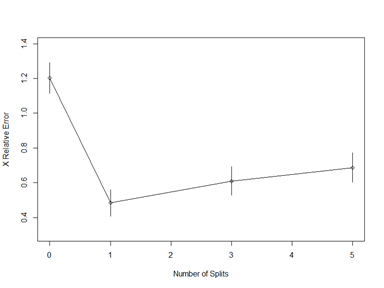Clearly, the tree should be pruned such that only the first split is retained.

```> printcp(rpart_fit2)
Classification tree:
rpart(formula = y_train ~ ., data = x_train, method = "class")

Variables actually used in tree construction:
 V11

Root node error: 64/130 = 0.49231

n= 130

CP nsplit rel error  xerror     xstd
1 0.54688      0   1.00000 1.20312 0.087545
2 0.10000      1   0.45312 0.48438 0.075918```

With this model, we can now check its fit with the training and, more importantly, the test datasets. The classification_summary function is a custom function that is included with the R code (provided as an appendix).

```> classification_summary(y_train_predict_label, y_train, 1, -1)
\$confusion_matrix
Predicted True Predicted False
Actual True              43              21
Actual False              8              58

\$misclassification_error
 0.2230769

> classification_summary(y_test_predict_label, y_test, 1, -1)
\$confusion_matrix
Predicted True Predicted False
Actual True              17              16
Actual False              6              39

\$misclassification_error
 0.2820513```

Thus, with rpart, we have a misclassification error of 0.2231 for the training data, and 0.2821 for the test data.

## 2. Random Forests

We begin this exercise by implementing random forests by setting the seed to 1 and using randomForest’s default parameters. The out-of-bag error is reported for the training dataset, and misclassification error for the test dataset.

Among these parameters, we are particularly interested in the mtry parameter, or the number of predictors to try at each split. By default, mtry is the square root of the number of parameters for classification problems. Because there are 60 feature variables in our present dataset, mtry is, by default, 7. To explore the effect of different seeds and mtry values, the out-of-bag error for the training dataset, and misclassification error for the test dataset, are reported at 3600 settings and plotted in heatmap and density distributions.

```> set.seed(1)
> rf_fit < - randomForest(x_train, y_train, mtry = floor(sqrt(ncol(x_train))))
> rf_fit
Call:
randomForest(x = x_train, y = y_train, mtry = floor(sqrt(ncol(x_train))))
Type of random forest: classification
Number of trees: 500
No. of variables tried at each split: 7

OOB estimate of  error rate: 19.23%
Confusion matrix:
-1  1 class.error
-1 57  9   0.1363636
1  16 48   0.2500000

> y_train_predict_label < - predict(rf_fit, x_train) # factor
> 1 - sum(y_train == y_train_predict_label)/length(y_train) # train error of 0
 0
> classification_summary(y_train_predict_label, y_train, 1, -1)
\$confusion_matrix
Predicted True Predicted False
Actual True              64               0
Actual False              0              66

\$misclassification_error
 0```

We observe that the out-of-bag error is 19.23% with a seed of 1 and mtry of 7. Interestingly, random forests fit the training set perfectly. There is no misclassification observed.

```> y_test_predict_label < - predict(rf_fit, x_test)  # factor
> 1 - sum(y_test == y_test_predict_label)/length(y_test) # test error of 0.1667
 0.1666667
> classification_summary(y_test_predict_label, y_test, 1, -1)
\$confusion_matrix
Predicted True Predicted False
Actual True              26               7
Actual False              6              39

\$misclassification_error
 0.1666667```

With the test dataset, we observe a 16.67% misclassification error. The class error for +1 is 21.2%, and 13.3% for -1. These class errors are comparable with the confusion matrix computed by random forests with the training set.

By changing the mtry number to 1 (or just one predictor tried at each split), we observe a smaller out-of-bag error of 16.15%. We also observe a 10.26% misclassification error with this mtry setting. This is interesting because I would have intuitively expected error to be larger!

```> set.seed(1)
> rf_fit < - randomForest(x_train, y_train, mtry = 1)
> rf_fit

Call:
randomForest(x = x_train, y = y_train, mtry = 1)
Type of random forest: classification
Number of trees: 500
No. of variables tried at each split: 1

OOB estimate of  error rate: 16.15%
Confusion matrix:
-1  1 class.error
-1 58  8   0.1212121
1  13 51   0.2031250

> y_test_predict_label < - predict(rf_fit, x_test)  # factor
> 1 - sum(y_test == y_test_predict_label)/length(y_test) # test error of 0.1667
 0.1025641
> classification_summary(y_test_predict_label, y_test, 1, -1)
\$confusion_matrix
Predicted True Predicted False
Actual True              28               5
Actual False              3              42

\$misclassification_error
 0.1025641```

What about an mtry of 2, 3, …, 60? Or seeds of 2, 3, …, n? Fortunately, we can ask R to compute the errors for all possible values of mtry, and a reasonable number of different seeds. With 60 mtry values (from 1 to 60, since there are 60 feature variables in the dataset) and 60 different seeds (from 1 to 60), we can obtain out-of-bag errors, and test misclassification errors, with 3600 different settings.

Levelplots, or heatplots, are particularly good at providing a general overview of trivariate data (seed, mtry, and error, in our case). 10×10 heatmaps are first provided, with errors labelled as text, to give a general sense of the range of errors over 100 settings. Then the 60×60 heatmaps are shown.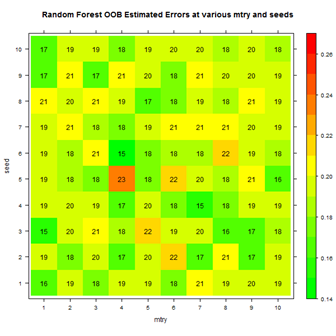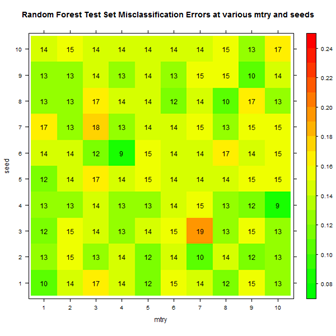Note that the color scale for errors is different for the two plots, since test classification error rates were generally lower than the OOB errors. Test error rates were also more variable, so the color scale for the plot on the right is wider than that for the plot on the left.

We see from the 10×10 heatmaps that out-of-bag errors can range roughly from 15% to 23%, and that the test set misclassification errors can range roughly from 10% to 19%. There are no patterns that are humanly discernible. For instance, there does not seem to be a relationship, so far, between mtry and error rates.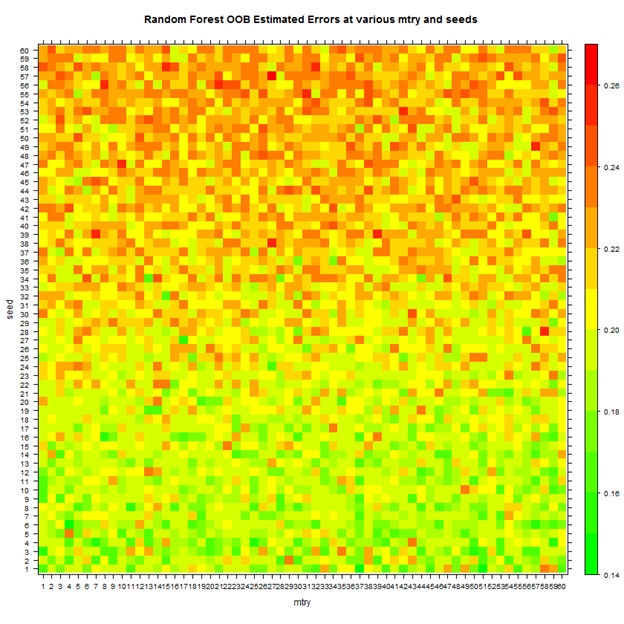The 60×60 heatmap shows a different picture. Surprisingly, error rates increase with the seed number. This is interesting because the seed number, to my knowledge, is pure for randomization purposes, and should not affect error rates substantially. However, at higher seed numbers, errors are almost double that of lower seed numbers.

Trying 60 seed numbers was an arbitrary decision (mostly for the aesthetic purpose of making a square heatplot). It would make more sense to try thousands, if not tens of thousands, of seed numbers to get the real picture. I suspect that there is actually no pattern. On the other hand, my knowledge of pseudorandom generators is very limited, and there very well might be a pattern.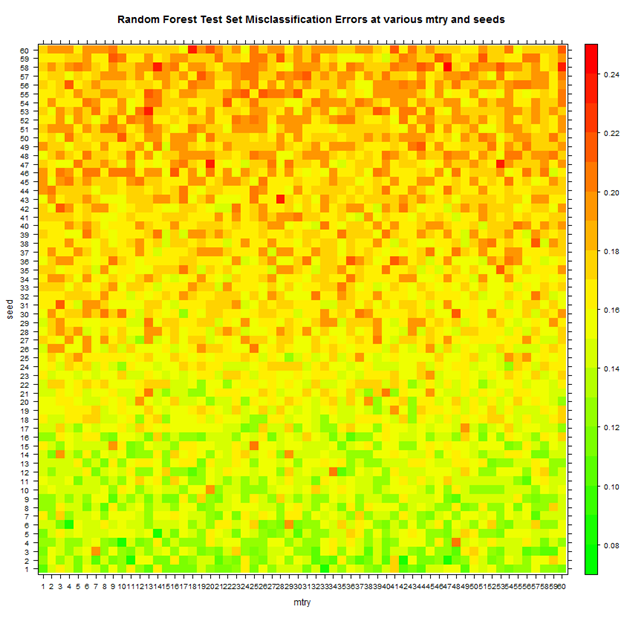We also observe the same apparent relationship between seed and error rate, with the test set. As with the 10×10 plot, there is no discernible pattern between mtry and error rate.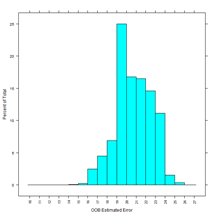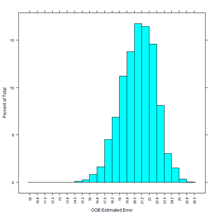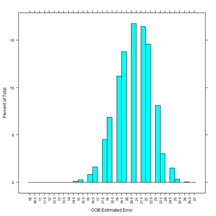The out-of-bound estimated error rates are plotted here as histograms. Bin size is decreased from left to right, from 0.0100 to 0.0075 to 0.0050. Interestingly, there is a slight left-skewedness evident in all three plots, with a mean (sd) of 0.2078 (0.0177) and median of 0.2077. At this time, I am uncertain on how to interpret this.

More interestingly, the right-most graph shows discontinuities in the distribution. Upon further investigation, it appears that many error rates are duplicated exactly, at different seeds. I believe this has to do with the bootstrap nature of random forests. With different seeds, the bootstrap procedure may be returning the same sample, or predictors, which lead to the same error rate. (An alternate explanation is that I may be running this “simulation” incorrectly.)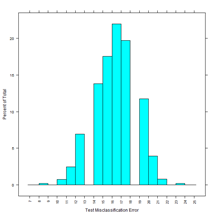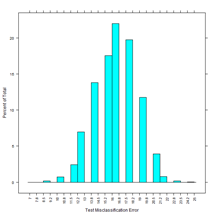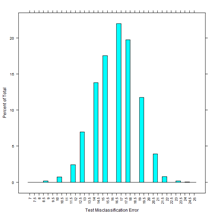We observe a more normal distribution with the test set misclassification error rates. The mean (sd) test error is 0.1639 (0.0228), and the median, 0.1667. We also observe the breaks in the distribution, which are more severe because there are fewer cases in the test dataset.

Is there a correlation between the out-of-bag error estimated by randomForest, and the actual misclassification error with the test dataset?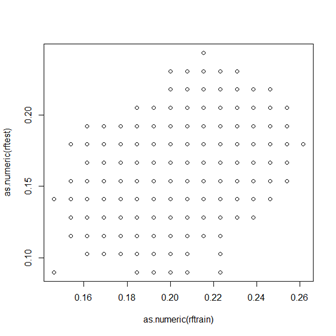The linear correlation coefficient r is 0.46. So there is some moderate linear relationship.

Adaptive boosting (“AdaBoost”) essentially iterates the same rpart algorithm from part 1. At the end of each iteration, errors are computed, and used to modify, or adapt, the subsequent iteration. This iteration reduces the instability of rpart, and makes AdaBoost less vulnerable to overfitting but more suspectible to noise and outliers, compared to rpart. As with random forests, there does not seem to be an outwardly random component (such as the bootscrap process used by random forests) that is affected by using different seeds.

The training and test errors can be plotted against iteration number: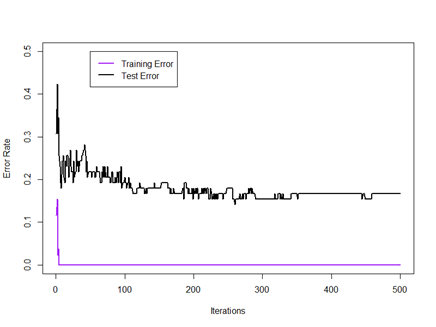We observe that errors at early iterations can fluctuate wildly, from between roughly 0.20 and 0.40 for the test error. However, with additional iterations, we observe the adaptive mechanism set in, to reduce and stabilize error rates. The final training error reached is 0, for a perfect fit. The final test error reached is 0.1667.

## 4. Table of Errors

This table briefly summarizes the training and test errors shown by each of the three procedures described previously. For rpart and AdaBoost, the misclassification errors from the training and tests sets are represented. For randomForest, the mean of the out-of-bag errors, and test set misclassification errors, computed at 3600 settings are represented (despite the slight skewedness of each distribution).

Note that this may not be the optimal method to evaluate randomForest, because typically only a range of mtry, or perhaps only the default mtry, will actually be used in practice. However, from the heatplots, we observed that changing mtry does not appear to substantially affect error rates.

 Training Error Test Error rpart 22.31% 28.21% randomForest 20.78% (OOB) 16.39% (sd = 2.28%) AdaBoost N/A (0.00%) 16.67%

References:

1. Fall 2012 UCLA Biostatistics 273 Lectures; Prof. Christina Kitchen
2. Stanford Statistics 202 Sample Code